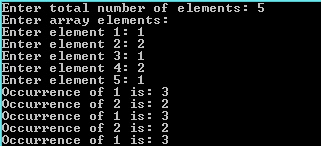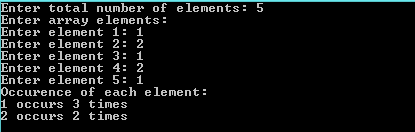# Find occurrence of each element in an array using simple method O(n^2) and hashing O(n) time

In this article, we are going to learn how to find occurrence of each element in an array using two different methods 1) simple method O(n^2) time and 2) hashing O(n) time with C programs?
Submitted by IncludeHelp, on December 08, 2017

It is the concept or logic used to make search operations faster i.e. Search an element in O(1) time. It treats the indexes of an array as the elements of another array.

Mainly it is used for the questions related to occurrence of elements of an array.

Let us understand it with the help of an example,

Given an array and we need to count of occurrence of each element.

Solution:

```/*C program to find occurrence of each element in one dimensional array.*/

#include <stdio.h>
#define MAX 100

int main()
{
int arr[MAX],n,i,j;
int num,count;

printf("Enter total number of elements: ");
scanf("%d",&n);

printf("Enter array elements:\n");
for(i=0;i< n;i++)
{
printf("Enter element %d: ",i+1);
scanf("%d",&arr[i]);
}

//count occurence of each element
for(i=0;i< n;i++)
{
count=0;
for(j=0; j<n;j++)
if(arr[i]==arr[j])
count++;
printf("Occurrence of %d is: %d\n",arr[i],count);
}

return 0;
}
```

OutputBut in this the Time Complexity is O(n^2) and it prints the occurrence statement for an element the no. of times it appears.

So, to solve this problem and reduce the complexity to O(n), we will use the concept of hashing.

## Using hashing

```/*
C program to find occurrence of each element in one dimensional array.
(Using hashing)
*/

#include <stdio.h>
#define MAX 100

int main()
{
int arr[MAX],n,i,j;
int num,count, hash[MAX] = {0};

printf("Enter total number of elements: ");
scanf("%d",&n);

printf("Enter array elements:\n");
for(i=0;i< n;i++)
{
printf("Enter element %d: ",i+1);
scanf("%d",&arr[i]);
}

// Using another arrays index as element
for(i=0; i<n; i++)
hash[arr[i]]++;

printf("Occurence of each element:\n");
for(i=0; i<n; i++)
{
if (hash[arr[i]]!=0)
printf("%d occurs %d times\n", arr[i], hash[arr[i]]);
hash[arr[i]] = 0;
}

return 0;
}
```

Output